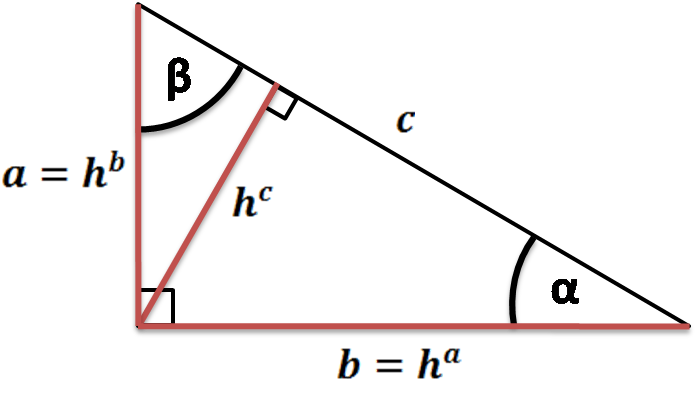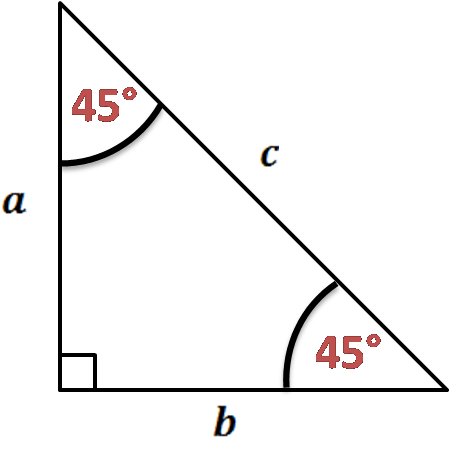# Area of a Right Triangle Calculator

Created by Hanna Pamuła, PhD candidate
Reviewed by Bogna Szyk
Last updated: Jun 26, 2020

If you are wondering how to find right triangle area, you're in the right place - this area of a right triangle calculator is a tool for you. Whether you're looking for the equation given triangle legs, leg and hypotenuse or side and angle, you won't be disappointed - this calculator has all of them implemented. Scroll down to find out more about area of right triangle formulas or simply give our calculator a try!

## Area of right triangle formulasThe basic equation is a transformed version of a standard triangle height formula (a * h / 2). Because the right triangle legs are perpendicular to each other, one leg is taken as a base and the other is a right triangle height:

area = a * b / 2

Sometimes it's not so obvious - you have other values given, not two legs. Then what?

1. If you have one leg and hypotenuse given, use the Pythagorean theorem to find the missing leg:

a² + b² = c²

Then take a square root of the transformed equation:

• given a and c we find that b = √(c² - a²):

area = a * √(c² - a²) / 2

• given b and c we calculate that a = √(c² - b²):

area = b * √(c² - b²) / 2

1. If you know one angle and hypotenuse, you can use the law of sines:

a = c * sin(α) b = c * sin(β) = c * sin(90-α) = c * cos(α)

area = c² * sin(α) * cos(α) / 2

2. Given one angle and one leg, find the area using e.g. trigonometric functions:

a/b = tan(α) and b/a = tan(β)

area = b * tan(α) * b / 2 = b² * tan(α) / 2

area = a * a * tan(β) / 2 = a² * tan(β) / 2

If you've just noticed that your triangle is not a right triangle, check out this general triangle area tool.

## Area of an isosceles right triangleIsosceles right triangle is a special right triangle, sometimes called a 45-45-90 triangle. In such triangle the legs are equal in length (as a hypotenuse always must be the longest of the right triangle sides):

a = b

One leg is a base and the other is the height - there is a right angle between them. So the area of an isosceles right triangle is:

area = a² / 2

## How to use the area of a right triangle calculator

Let's show the step by step calculation:

1. Pick one option, depending on what you have given. Assume that we know one leg and angle, so we change the selection to given angle and one side.
2. Enter the values. For example, we know that α = 40° and b is 17 in.
3. Watch our area of a right triangle calculator performing all calculations for you! The area of the chosen triangle is 121.25 in².
Hanna Pamuła, PhD candidate
Given
two sidesa
in
b
in
c
in
Area
A
in²
People also viewed…

### Cross multiplication

The cross multiplication calculator is here to help you solve all the math problems involving an equation between two fractional expressions.

### Discount

Discount calculator uses a product's original price and discount percentage to find the final price and the amount you save.

### Divisibility test

Become a master of testing for divisibility with the help of our divisibility test calculator!

### Steps to calories

Steps to calories calculator helps you to estimate the total amount to calories burned while walking.